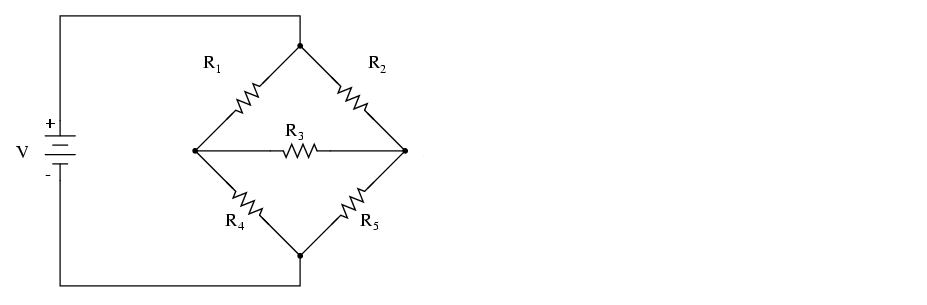# Problem: In the following circuit,V= 25 V, R 1 = 5 Ω, R2 = 15 Ω, R3 = 25 Ω, R4 = 10 Ω, and R5 = 20 Ω. Find each current in the circuit.

###### FREE Expert Solution
90% (272 ratings)
###### Problem Details

In the following circuit,V= 25 V, R 1 = 5 Ω, R2 = 15 Ω, R3 = 25 Ω, R4 = 10 Ω, and R5 = 20 Ω. Find each current in the circuit.